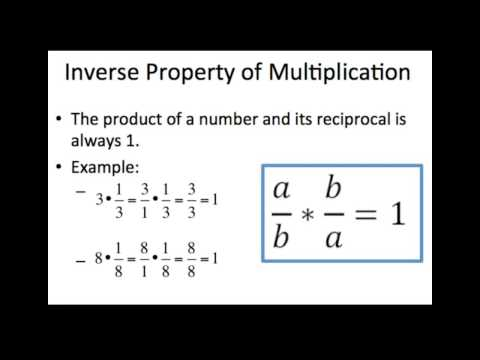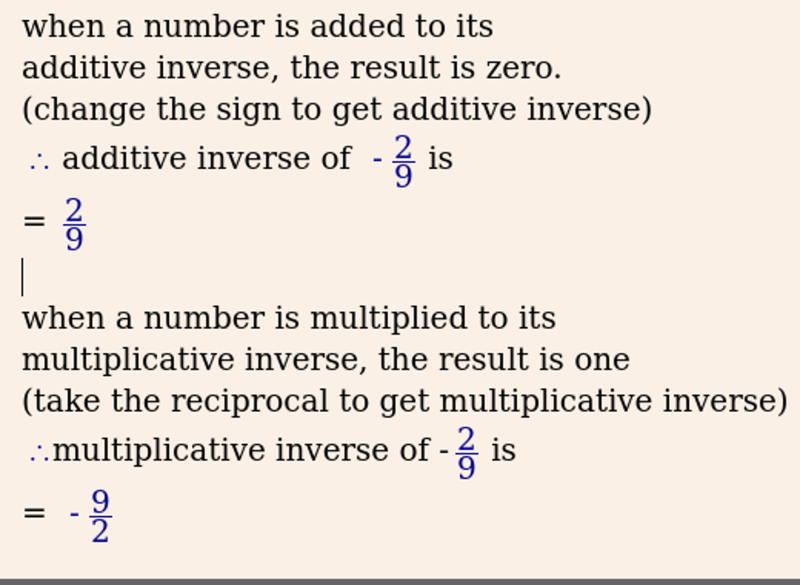# Inverse Property of Numbers – Definition, Formula and Solved Examples

We will aim to understand the definition and formula of inverse property in this lesson. Its principles can be used to find additive inverse as well as multiplicative inverse in mathematical equations. This is best understood with the help of examples.

## Inverse Property of Rational Numbers

Several types of properties apply to rational numbers. Firstly, rational numbers are numbers that can be expressed in the form of x/y. This is where both ‘x’ and ‘y’ are integers. Here, y is not equal to 0. Integers that can be represented on the number line are called rational numbers.

The general properties apply to this category of numbers. They are mainly – associative property, distributive property, commutative property, and identity property. One of these is called the inverse property. The inverse property of rational numbers basically undoes each other. The two types of inverse property – Additive Inverse Property and Multiplicative Inverse Property have been discussed in this lesson.When the sum of two real numbers is zero, then each real number is the additive inverse of the other. It is synonymous with terms such as opposite number, sign change, and negation. The simple rule is to change the positive number to a negative number and vice versa to obtain the sum of zero. This is useful for getting rid of an equation’s terms.

Z+(-Z) = 0, where Z is a Real number. The additive inverses of each other are Z and -Z.  For example, 12+ (-12) =0. Thus -12 is the additive inverse of 12 and 12 is the additive inverse of -12.

This property is valid for real numbers. This includes whole numbers, natural numbers, integers, fractions, decimals, or any real number. The negative of any given number is its additive inverse. It is important to remember that the additive inverse of zero is zero.

Let us consider the case of algebraic expressions. The additive inverse of an algebraic inverse will make the sum of all the terms zero. Hence, the additive inverse of the expression is -(expression). The additive inverse of y2 + 1 is – (y2 + 1) = -y2 – 1.### Formula of Additive Inverse Property

Additive Inverse of R = –1 × (R)

## Multiplicative Inverse Property

A multiplicative inverse is in essence, a reciprocal. A reciprocal is one of a pair of numbers that when multiplied with another number equals 1. Similarly, when the product of two numbers is 1, they are said to be multiplicative inverses of each other. The inverse property of multiplication states that the product of a number and its multiplicative inverse is 1. This is the identity element for multiplication. It is useful for getting rid of fractions.

The multiplicative inverse of ‘s‘ is denoted by s-1 or 1/s. For example, 5 x 1/5 = 1. This is why 1/5 is the multiplicative inverse of 5. Likewise, the multiplicative inverse of 1/130 is 130.

This property is valid for natural numbers, integers and fractions. In the case of natural numbers, the multiplicative inverse of that number ‘n’ is 1/n. 7 is a natural number. If we multiply 8 by 1/8, the product is 1. Therefore, the multiplicative inverse of 8 is 1/8.

We will now extend our understanding to negative natural numbers. The multiplicative inverse of any negative number is simply its reciprocal. For example, (-7) × (-1/7) = 1, therefore, the multiplicative inverse of -7 is -1/7.### Formula of Multiplicative Inverse Property

To summarise, the multiplicative inverses are as follows:

 Type Multiplicative Inverse Example Natural Number x 1/x Multiplicative Inverse of 9 is 1/9 Integer x where x ≠ 0 1/x Multiplicative Inverse of -2 is -1/2 Fraction a/b; a,b ≠ 0 b/a Multiplicative Inverse of -13/9 is -9/13

## Important Tips on Multiplicative Inverse Property

• It is possible to obtain the multiplicative inverse of a fraction. The reciprocal must be calculated. Flip the numerator and denominator for this.
• The multiplicative inverse of 1 is 1 while for 0, it is not defined.
• The multiplicative inverse of a mixed fraction is obtained by converting the mixed fraction into an improper fraction. Furthermore, determine its reciprocal.

## What is the difference between additive inverse and multiplicative inverse?

An additive inverse is a number added to an equation to make its sum zero. In contrast, the multiplicative inverse is the reciprocal of a given number. It gives the product as 1 when multiplied.• What is the additive inverse of 2/3?

We know that the given number and its additive inverse’s sum should be 0.

Let x be the additive inverse.

2/3 + x = 0

x = -2/3

Hence, the additive inverse of 2/3 is -2/3.

• Solve for Q using the Additive Inverse Property.

Q + 5 = 8

We have to remove the +5. The inverse property of addition can be employed and -5 can be applied to both sides to get rid of it. -5 is the additive inverse ( 5 – 5 = 0). It is added to each side of the equation.

Therefore,

Q+5 -5 = 8 – 5

Q = 3.

Hence, Q = 3.

## Multiplicative Inverse Property Example

• What is the multiplicative inverse of 1/5? Also, verify your answer.

The multiplicative inverse of 1/5 is 5/1.

We know the given number and its multiplicative inverse’s product should be 1.

To verify it, we will multiply 1/5 with its multiplicative inverse.

(1/5) × (5/1) = 1.

Hence, the multiplicative inverse of 1/5 is 5/1.

• Solve for X in the following equation using the inverse property of multiplication.

7X = 42

The multiplicative inverse property of the number positive natural number 7 can be used. This is to eliminate the +7 of the 7X. As we know, (7) x ( 1/7 ) = 1.

Proceed to multiply each side of the equation by the multiplicative inverse = 1/7.

Therefore,

7X * 1/7 = 42 * 1/7

X = 6.

Hence, X= 6.

• What is the reciprocal of 121/11?

At first glance, the reciprocal of 121/11 seems to be 121/11.

We get the following on further simplification:

121/11 = 11/1.

Furthermore, 11/1 × 1/11 = 1 satisfies the property of multiplicative inverse

Therefore, the reciprocal of 11/1 is 1/11.